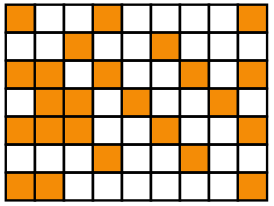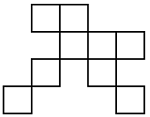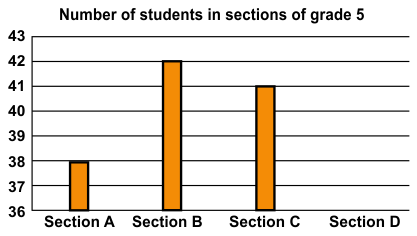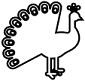# CREST Mathematics Olympiad Class 5 Sample Papers

## Syllabus:

Section 1: Numerals, Number Names and Number Sense (7 and 8 digit numbers), Computation Operations, Fractions and Decimals, Measurement of Length, Weight, Capacity, Volume, Time, Temperature and Money, Conversions, Geometrical Shapes and Solids, Angles, Perimeter of Various Shapes & Area of Rectangle and Square, Symmetry, Data Handling.

Achievers Section: Higher Order Thinking Questions - Syllabus as per Section 1

 Q.1 How many more blocks need to be shaded in the given figure to represent a fraction of 3/7?Q.2 Solve the following: 4 1/4 + 12/5 + 5.75 - 8.25
 Q.3 If the side of each square in the given figure is 8 m, then find the area and the perimeter:Q.4 A rectangle measuring 12 cm x 84 cm is divided into 7 parts such that each part is a square. Find the area of 3 of these parts:
 Q.5 Arrange 0.34 km, 56000 cm, 310000 mm and 1600 m in descending order:
 Q.6 Find the value of the following: [823 + (-398)] + [413 - 312 + (-400)] - (-30) + 286 - {(-115) + 117}
 Q.7 If the total number of students in four sections of grade 5 is 160, then find the number of students in section D:Q.8 Where will the hour hand of a clock stop if it shows 8 o'clock and turns one right angle clockwise?
 Q.9 If A*B = AB(A - B), then find the value of 509*218:
 Q.10 Which of the given options show how the given figure would like if it is quarterly rotated in the clockwise direction?Sample PDF of CREST Mathematics Olympiad for Class 5: